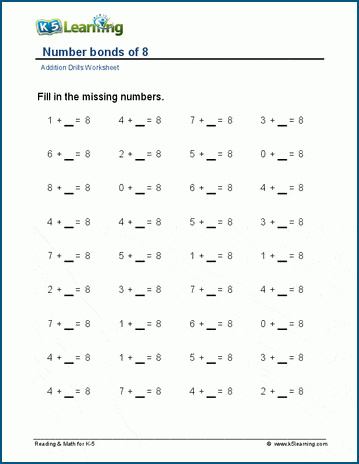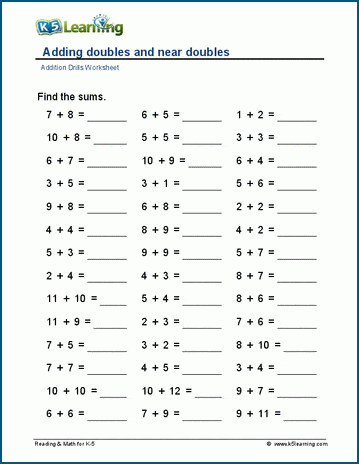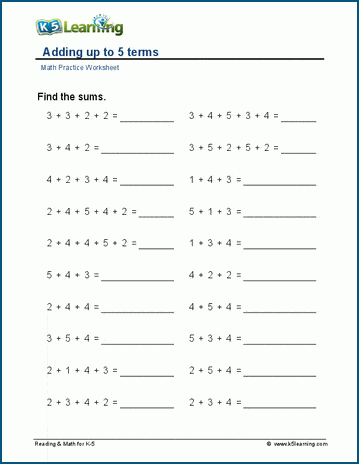# Basic Addition Math Drill Worksheets

We start the basic addition facts with a series of addition facts tables, from 0 – 5, 1 – 10, and 1 – 12, with and without answers.

## Addition tables practice in order

These tables display all the addition facts 1 – 10, and 1 - 12 in order. Each worksheet has less and less hints, making each one harder to fill in.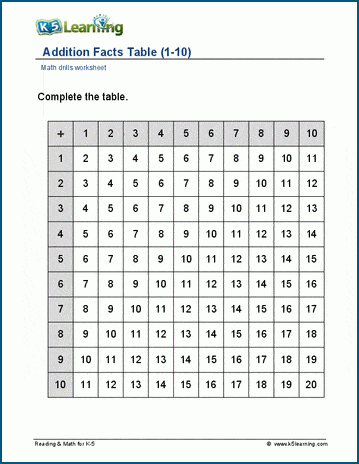## Addition tables practice in random order

The next few sets of worksheets present the addition tables in random order 1 – 10, and 1 – 12, with hints and without. This is what the addition table 1 – 12 with less hints looks like.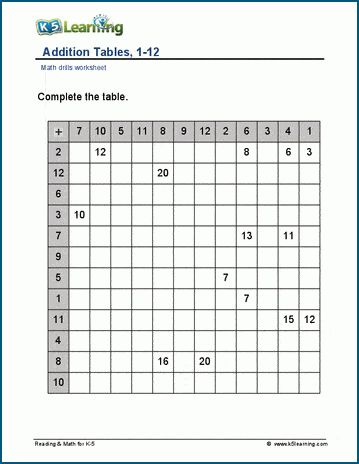## Worksheets to practice basic addition facts vertically

The next area presents worksheets to practice the addition facts in the vertical format, starting within 5, then within 10 and finally within 12. This worksheet contains 100 questions per page, which may prove suitable for timed practice.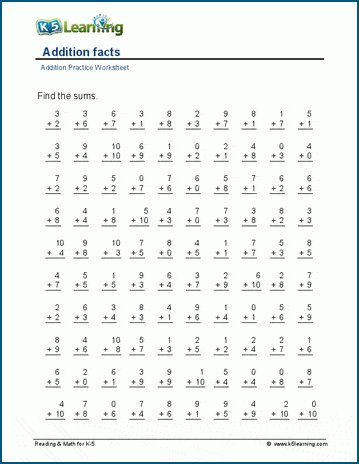Similar to the above area, the five pages of worksheets in this area has students practice adding numbers horizontally, including within 5, within 10, and within 12. This worksheet displays addition facts with addends of 1 – 10 in the horizontal format.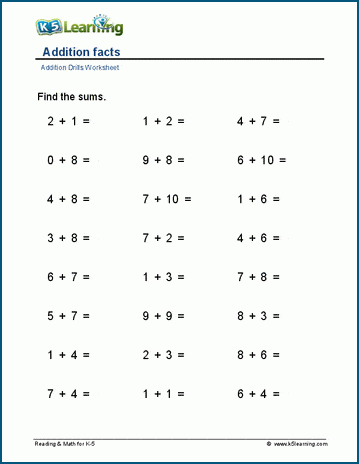Students work with missing addends in this section, starting within 0 – 5, then sums under 10 and 20. The final two pages contain worksheets where the sums are 9 and then 10. Here’s what the worksheet looks like for making sums of 9.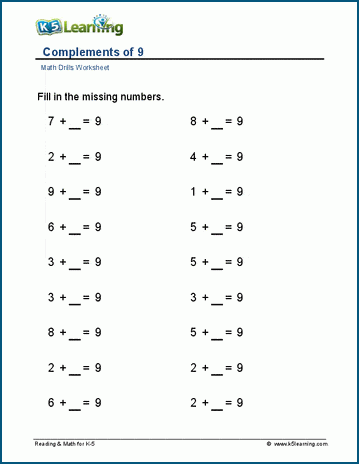In the next section, worksheets cover adding zeros to numbers, adding ones to numbers, adding twos to numbers, etc. up to 10. As an example, here’s the adding fours to numbers worksheet.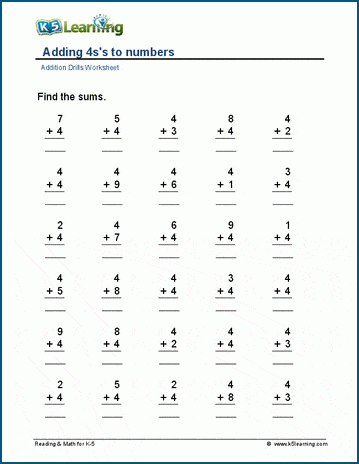## Circle drills

There are two separate sets of circle drills: 0 – 5 and 1 – 10. Here’s one of the 1 – 10 circle drill worksheets.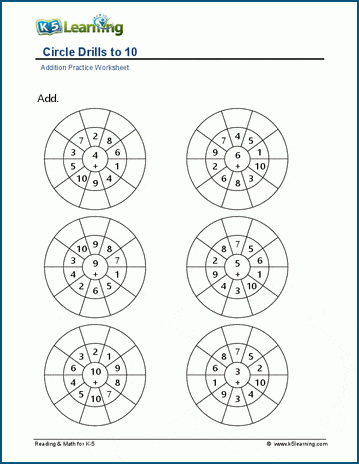## Number bonds practice

Next, we have number bonds worksheets for basic addition. They are segmented by 5/6/7/, 8/9/10, 11 – 15, and 16 – 20. This is one of the 8,9, and 10 number bonds worksheets.